# Mathematics

### Circle Theorems

There are eight circle theorems. They are as follows:

• Circle Theorem 1: The angle subtended by a chord at the centre is twice the size of the angle subtended by the same chord at the circumference.
• Circle Theorem 2: The angles subtended by a chord in the same segment are equal.
• Circle Theorem 3: The angle of a semi-circle is a right angle.
• Circle Theorem 4: The opposite angles of a cyclic quadrilateral add up to 1800.
• Circle Theorem 5: The angle between a tangent and a radius at the point of contact is a right angle.
• Circle Theorem 6: The two tangents drawn to a circle from a point are equal in length.
• Circle Theorem 7: The angle between a chord and a tangent is equal to the angle subtended by the same chord in the alternate segment.
• Circle Theorem 8: The perpendicular line from the centre to a chord, bisects it.

The following image shows the basics of a circle; they are really important to learn the circle theorems.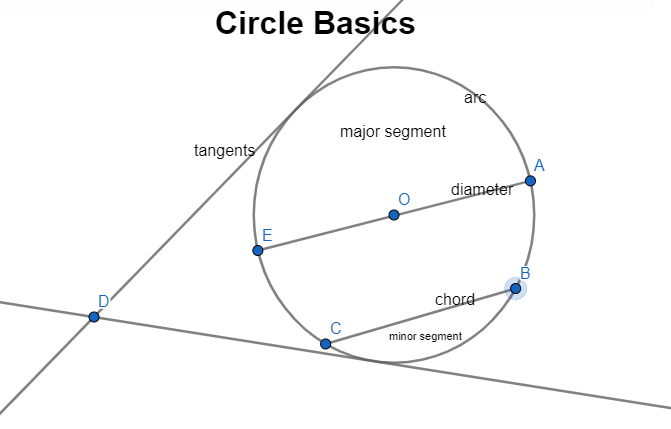You can practise the seven circle theorems with the following simulations; just move the point/s on the circumference and see how they work for yourself. It's fun!

Amazon Best Sellers:

These flash cards will make a significant difference when you revise for your forthcoming exams: very informative and neatly presented; they became best sellers for a reason.AS and A Level Only

#### Circle Theorems - proof

The following images show how circle theorems can be proven from basic geometry. Most examination boards in England want students to learn the proofs along with the theorems.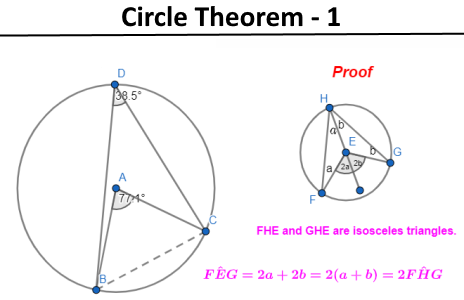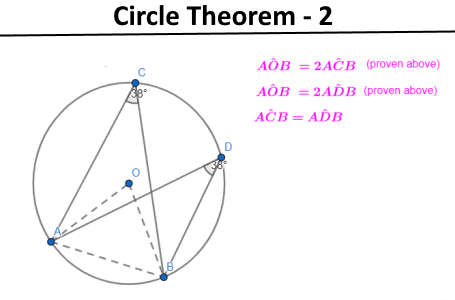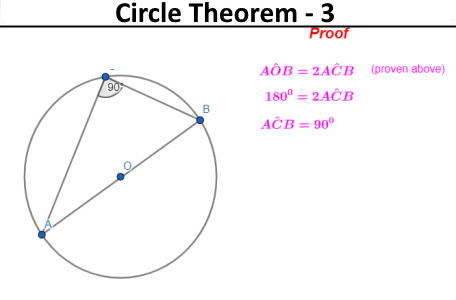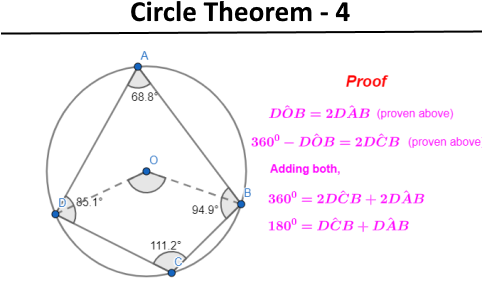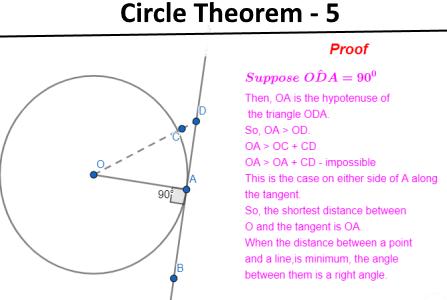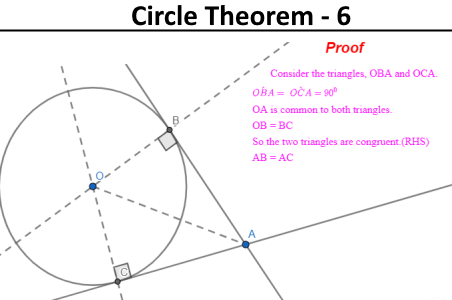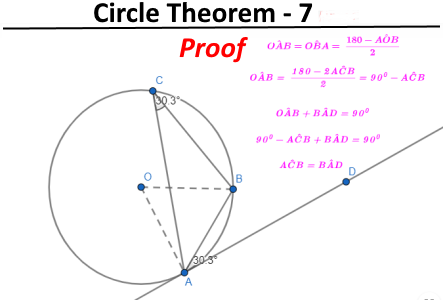#### Problem Solving - circle theorems

E.g.1

If BC = CD and ∠DCF = 270 Find ∠BAC and ∠BCE.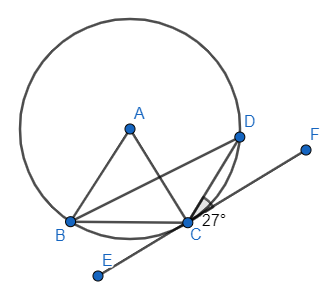∠CBD = 270 (alternate segment theorem)
∠BDC = 270 (isosceles triangle)
∠BAC = 540 (angle subtended by a chord at the centre = 2 x angle subtended by a chord at the circumference)
∠BAD = 180 -54 = 1260 (angle of a triangle add up to 1800)
∠BCE = 180 -(126+27) = 27 (angles on a straight line add up to 1800)

E.g.2

If BA = x, AC = (x - 3), AD = 3cm and EA = 6cm Find x.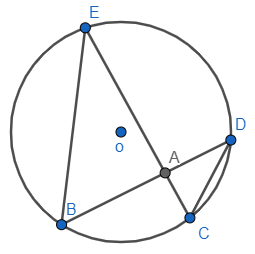∠BEA = ∠ADC (angles subtended in the same segment)
∠EBA = ∠ACD (angles subtended in the same segment)
∠EAB = ∠DAC (vertically opposite angle)
So, EAB and DAC triangles are similar.
BA/AD = EA/AC (corresponding sides are in the same ratio)
x(x - 3) = 6 x 3
x2 - 3x - 18 = 0
x = 6 or x = 3.
x = 6cm.

E.g.3

Show that BE is parallel to CO.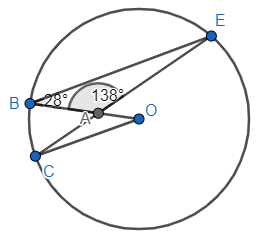∠BEC = 180 - (28 + 138) = 140 (angles of a triangle)
∠BOC = 280 (angles subtended by a chord at the centre is twice that of the same at the circumference)
∠CAO = ∠BAE (vertically opposite angle)
∠ACO = 180 - (28 + 138) = 140 (angles of a triangle)
∠BEA = ∠ACO and ∠ABE = ∠AOC (appear to be alternate angles)
So, BE//CO.

E.g.4

Find ∠EDC, ∠DFE and ∠ECD.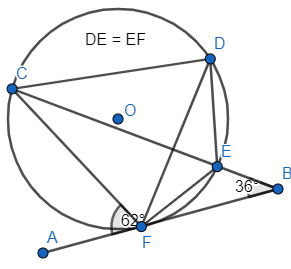∠CFB = 180 - 62 = 1180 (angles on a straight line)
∠FCB = 180 - (36 + 118) = 260 (angles of a triangle)
∠FDE = ∠FCB (angles subtended by the same chord in the same segment)
∠FDC = 620 (alternate segment theorem)
∠EDC = ∠FDC + ∠FDE = 62 + 26 = 880
∠DFE = ∠FDE = 260 (FED is an isosceles triangle)

E.g.5

Find ∠AOB.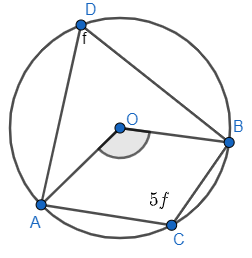5f + f = 180 => 6f = 1800
f = 300
∠AOB = 2 x ∠ADB (angles subtended by a chord at the centre is twice that of the same at the circumference)

∠AOB = 600

Challenge

Show that FEGH is a cyclic quadrilateral.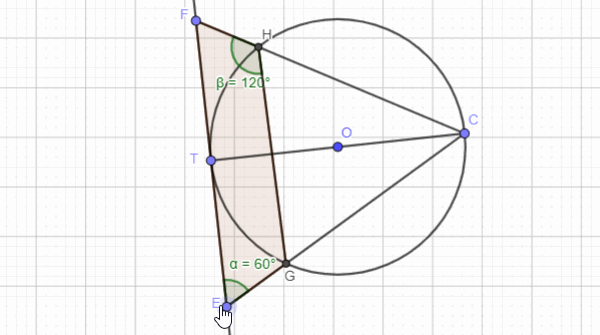Maths is challenging; so is finding the right book. K A Stroud, in this book, cleverly managed to make all the major topics crystal clear with plenty of examples; popularity of the book speak for itself - 7th edition in print.

### Recommended - GCSE & iGCSEThis is the best book available for the new GCSE(9-1) specification and iGCSE: there are plenty of worked examples; a really good collection of problems for practising; every single topic is adequately covered; the topics are organized in a logical order.

### Recommended for A LevelThis is the best book that can be recommended for the new A Level - Edexcel board: it covers every single topic in detail;lots of worked examples; ample problems for practising; beautifully and clearly presented.